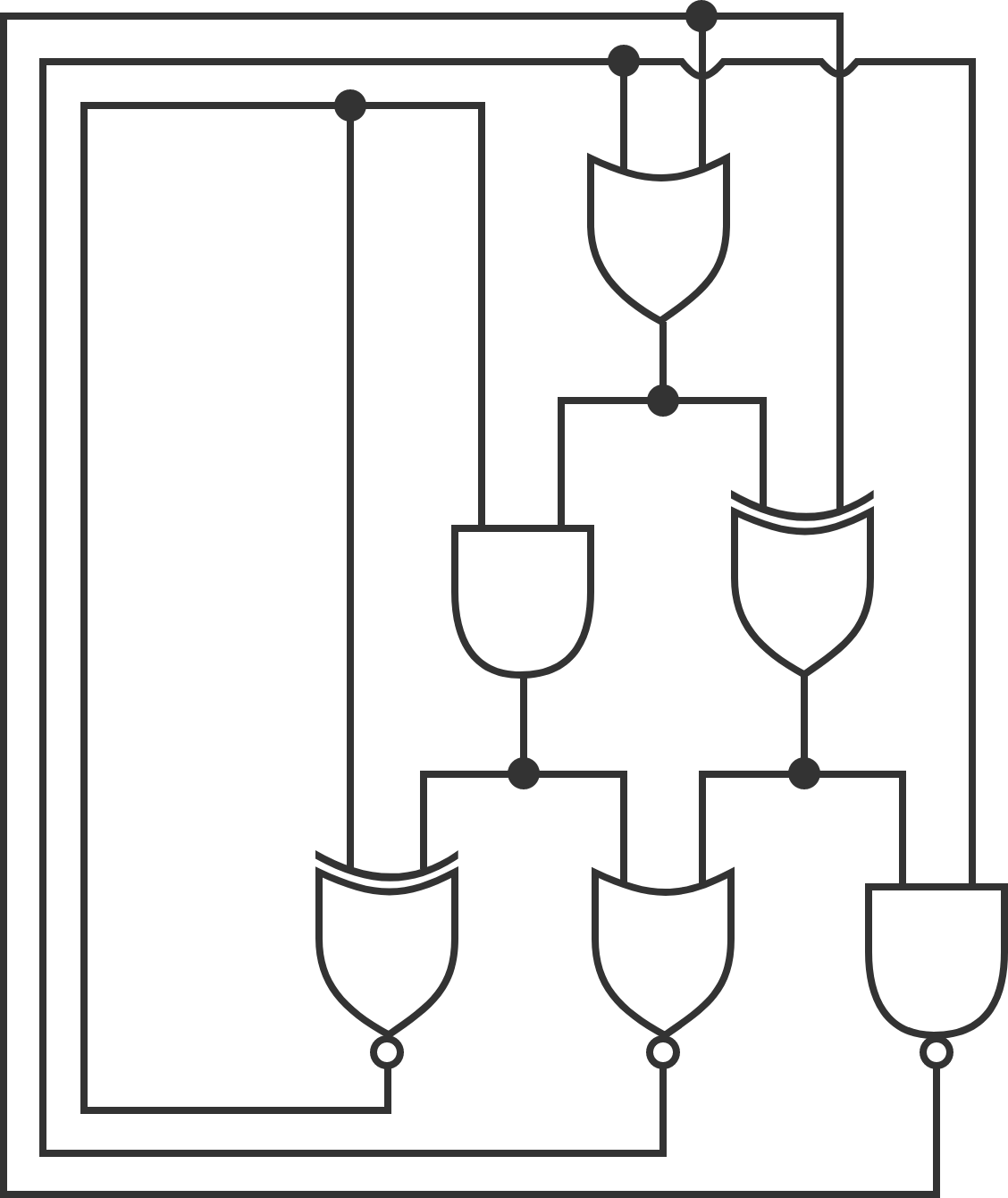# Self-referential circuit

Logic Level 3The above is a logic circuit, made up of logic gates. Each wire holds either the value true or the value false. A black dot indicates that the wires meeting at that point have the same value. The six big things are logic gates; each receives two inputs from the top side and produces a value at the bottom. The gates can be seen in the above page; note that the three bottom gates have a white dot at the bottom (their outputs are inverted).

Only one configuration of the logic circuit shown is stable. Determine this configuration. Enter your answer as a 6-digit binary number representing the output of each gate (starting from the single gate on the top row, then the two on the middle row from left to right, then the three on the bottom row from left to right), where 1 = true and 0 = false. (Leading zeros are ok.)

×